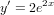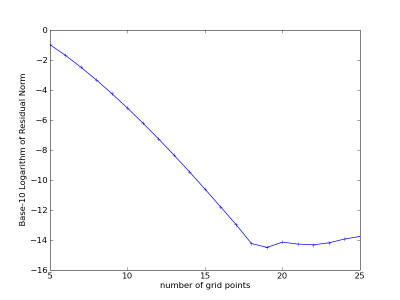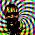## Saturday, May 30, 2009

### FFTW Discrete Cosine Transform Derivative

I've done several posts lately about finite difference methods. Spectral methods are the ultimate extension of these same basic ideas used from Boole on up to the present. For an interesting read on this progression (or lack thereof) check out the preface of Boyd's spectral methods book.

Instead of using a series of overlapping local derivative expressions, the derivative at each point is calculated with an interpolating polynomial that spans the entire grid. This leads to exponential (rather than the usual first, second, etc) order of convergence. For folks used to finite differences, Fornberg has written a concise guide that focuses especially on the connection between finite difference approximations and pseudospectral methods.

There are a couple of things that make spectral methods practical for a wide variety of engineering problems (not just periodic boundaries). The most important being Chebyshev Polynomials, which through a little change of variables can be calculated with Fourier transforms, or discrete cosine transform (DCT) for a factor of four speed-up over the FFT. Luckily the excellent FFTW library has specialized transforms for real, even data which we can use to calculate our DCTs with nice, optimized O(n*log(n)) algorithms for arbitrary length data. Here is a thread discussing the usage of FFTW's DCT for calculating derivatives.

Chebyshev polynomial in terms of cos():From the FFTW docs the forward and inverse discrete cosine transforms (DCTs) areNotice the similarity between the Chebyshev polynomial and the formula for the coefficients of the DCT.

Here's a Fortran routine that uses the DCT Type II and Type III from FFTW to calculate the derivative of a non-periodic function. An important thing to do when using spectral methods on non-periodic functions is to choose grid points that are at the roots of the Chebyshev polynomials. This makes them equally spaced in the angular coordinate, so we can use the standard transform libraries (which expect equally spaced data). Choosing nodes this way also helps with Runge's Phenomenon.

subroutine dct_diff(dydx, y, half_interval, n)
! use the discrete cosine transform from fftw to calculate the derivative
integer, intent(in) :: n ! number of data points
double precision, intent(in), dimension(n) :: y ! function values
double precision, intent(out), dimension(n) :: dydx ! derivative values
double precision, intent(in) :: half_interval ! half the interval length
double precision, dimension(n) :: beta ! derivative coefficients
double precision, dimension(n) :: alpha ! function coefficients
integer :: plan1, plan2, i
integer :: n_logical ! the logical size of the transform, size of
! the corresponding real symmetric DFT

! the logical size depends on the type of transform, check the docs:
! http://www.fftw.org/doc/1d-Real_002deven-DFTs-_0028DCTs_0029.html
n_logical = 2*(n)

! forward DCT:
call dfftw_plan_r2r_1d(plan1, n, y, alpha, FFTW_REDFT10, FFTW_ESTIMATE)
call dfftw_execute_r2r(plan1, y, alpha)
call dfftw_destroy_plan(plan1)
alpha = alpha / half_interval

! recurrence for the derivative coefficients:
beta(n) = 0D0
beta(n-1) = 2D0 * dble(n-1) * alpha(n)
do i = n-1, 2, -1
beta(i-1) = beta(i+1) + 2D0 * dble(i-1) * alpha(i)
end do
beta = - beta ! this makes it work, but not sure why!

! inverse DCT:
call dfftw_plan_r2r_1d(plan2, n, beta, dydx, FFTW_REDFT01, FFTW_ESTIMATE)
call dfftw_execute_r2r(plan2, beta, dydx)
call dfftw_destroy_plan(plan2)

! FFTW computes the un-normalized transforms, normalize by logical size
dydx = dydx / dble(n_logical)

end subroutine dct_diff

I compile this with F2Py so I can use all the nice facilities of Numpy and Scipy. Here's a Python convenience function for giving nodes based on the Chebyshev roots:

def arb_int_opt_nodes(a,b,n): # function to give Chebyshev points on an interval
y = numpy.zeros(n)
for i in xrange(n):
y[n-i-1] = numpy.cos( (2*i + 1)*numpy.pi/(2*n) )
return(((b - a) * y + b + a) / 2.0)

Try it out on a test function:with a known analytical derivative:The test function and its derivative using six grid points:Convergence graph:Pretty awesome, down to the round-off plateau with less than 20 points on the interval. This recent book on Chebyshev polynomials has a good tabulated example of integrating and differentiating a Chebyshev series on pp 33 - 35 that was very useful in debugging.

Perhaps a more practically useful test function is one-wavelength of cos(). This will give us an idea of how many nodes per wavelength we need for high accuracy solutions.Which looks to give a rule of thumb of about twenty nodes per wavelength to get down to the round-off plateau (32 bit double precision).

Scroll back up and take a look at the Fortran again, see that sign change after the recurrence? Know why it's there? Leave a comment and let me know if you think you do.

1.Hi jstults,

thanks for your blog ... it really helps a lot.
I am still working on figuring out all details of chebyshev ...

in arb_int_opt_nodes(..) you define the chebyshev-nodes at
y[0..n-1] = cos(pi) .. cos(pi/(2n))
shouldn't that be at
y[0..n-1] = cos(pi-pi/(2n)) .. cos(pi/(2n)) for
REDFT10 ?

Greetings
Jens

2.sorry... arb_int_opt_nodes(..) is correct and returns
y[0..n-1] = cos(pi-pi/(2n)) .. cos(pi/(2n))

----
Because REDFT10 is shifted by j+0.5 (j[0..n-1]) the chebyshev-nodes of the input data are set at
y[j=0..n-1] = +cos(pi -pi/n*(j+0.5))
= -cos(pi/n*(j+0.5))
= -cos(pi/(2n) *(2j+1))
You are using
y[n-i-1] = cos((2i+1)*pi/(2n)), i[0..n-1]
which is the same result.

Greetings
Jens

3.Hi Jens,

Glad it's helpful; you also may want to look at Calculating Derivatives of Unequally Spaced Points, you don't have to use a Chebyshev-roots grid (but it is optimal).

4.the last post also explains the "beta=-beta"-problem you have:
because y[0..n-1]=-cos(pi/(2n) *(2j+1) this minus-sign should go into x of cos(n*arccos(x)) and therefor into Xj of the DCTs.

Greetings
Jens

5.Good catch Jens, thank you.

6.Hi jstults,

for computational fluid dynamics (CFD) I am calculating a lot on 3D-grids with periodic boundaries in all directions using the lib "p3dfft".
Calculating derivatives for this is easy, because you can do 3d-fft. Multiplying the 3d-result in fourier-space with a certain fixed 3d-array and transforming it back to physical space returns the derivative in x,y or z.

Now my question:
I would like to change the boundaries that I only have periodicity in x and y but not in z (calculating channel flows). Therfor I want to use chebyshev-transform in z.
Is it possible to recive derivatives by forward-transform a 3d-array(physical space) to a 3d-array(fourier space) using r2r_cheby(in x) than r2c_fourier(in y) than c2c_fourier(in z). I wonder if it is "legal" to mix 2xDFT and 1xDCT and how to modify the resulting complex-data to get derivatives after backward-transform.

7.Jens: using the lib "p3dfft"
Why not fftw? Is there something you especially like about 'pd3fft' (I'm always curious about exploring options)?

I have been meaning to try a similar project for a flat plate boundary layer study (so it would be DCTs in stream-wise and wall-normal, and FFT's span-wise).

I haven't actually done it yet though, so I couldn't give you any practical implementation suggestions or pitfalls. If I recall correctly (it's been a while) Boyd describes a mixed approach like this applied to a global circulation model in his book (but doesn't go into much detail). If you find any good links please share, sorry I couldn't be of more help, and good luck!

8.Oh, I see, p3dfft is an MPI/Fortran wrapper around other FFT libraries like FFTW, nice.

9.p3dfft does the decomposition+3dfft of a 3d-grid over thousands of CPUs ...
internal it does the following:
state: all cpus have complete lines of real-data (phys.space) in x-direction
1) 1d-fft of all x-lines on all cpus (r2c)
2) rotate data that all cpus have complete lines of data in y-direction
3)1d-fft of all y-lines on all cpus (c2c)
4)rotate data that all cpus have complete lines of data in z-direction
5)1d-fft of all z-lines on all cpus (c2c)
=> 3d-fft with distributed memory (tested in my case with 16k cpus on BlueGene/P)

I found a GPL-code for 3d-channelflow on www.channelflow.org.
It combines fourier+chebyshev+fourier and does an differentiation(REDCT00) using this transformed data.
The following functions describe all this:
FlowField.h
void FlowField::makeSpectral()
void FlowField::makePhysical()
diffops.h
FlowField diff(..)
FlowField xdiff(..)
FlowField ydiff(..)
FlowField zdiff(..)

I would like to extend p3dfft with that feature.

10.Hi,

another comment ;)
I added the fourier-fourier-chebyshev to p3dfft and finally found the real reason for your beta=-beta problem.
It is actually quite simple, but important to know:

I used REDFT00 for which FFTW expects to chebyshev-nodes to be calculated with:
Fortran: coordZ(k) = cos(pi*(k-1)/(n3-1))
C++ : coordZ(k) = cos(pi*k/double(n3))
=> coordZ needs to run from +1 to -1 (not -1 to +1)

I suppose FFTW expects the coordinates to run from +1 to -1 for REDFC01, too.

Greetings
Jens

11.Thank you again Jens, it's nice to get all these little gotchas up for people to learn from. These down in the code details don't tend to make it into text books (but they tend to be a big stumbling block for learners).

12.Hi jstults,

I found the following sentence in "Spectral Methods" by C.Canuto in chapter 2.4.1:
"Virtually all of the classical literature on Chebyshev spectral methods uses this reversed order. Therefore, in the special case of the Chebyshev quadrature points we shall adhere to the ordering convention that is widely used int the literature (and implemented in the available software)."

Greetings
Jens

13.You might be interested in the fact that your post got copied over here. Looks like a farm anyway.

http://onlinegeometryproblems.blogspot.com/2010/08/fftw-discrete-cosine-transform.html

Oh and by the way: Very informative post - doing something similar myself atm.

cheers

14.Thanks Anonymous; I filed a TOS complaint with Blogger against that farm (it's funny, they even kept the links to my previous posts intact).

15.Great Blog... thanks for helping out the lot of us wandering about details of implementation (and guts) of these methods... Keep posting, please!!!

16.Great blog! I am trying to compute laplacian of a 2D function (non-periodic) using FFT technique. I first tested periodic functions and things work just fine. I am trying to extent your example to 2D function but cannot seem to figure our the recurrence for the derivative. My trial function is exp(x+y) sampled on grid with Nx and Ny points along x and y axis. The analytical result is 2.0*exp(x+y). I can send you my code if you want to see - but I have no luck so far. Any clues? Please help. Thx Amit

1.Hi Amit,
It's hard to tell what might be going wrong with your code without more details. What grid point distribution are you using? For your periodic tests you probably used evenly spaced points. Are you using a Chebyshev roots grid for your non-periodic tests?

2.See the sections in Chapter 6 of Boyd's book with the heading "Special Problems in Higher Dimensions:" for some pointers that might be helpful. Good luck with your calculation; would love to see your results when you get them.

3.